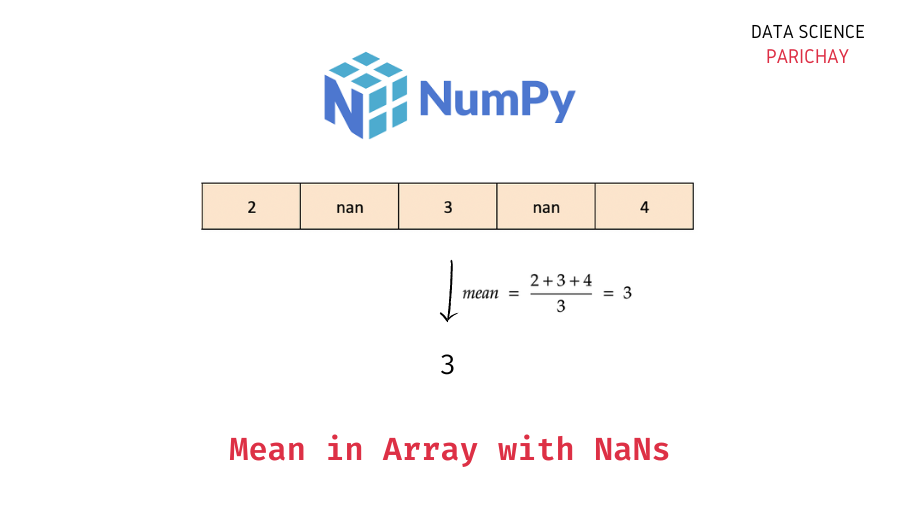# Mean of Numpy Array with NaN Values

The Numpy library in Python comes with a number of useful built-in functions for computing common descriptive statistics like mean, median, standard deviation, etc. In this tutorial, we will look at how to get the mean value of a Numpy array containing one or more NaN values.

## Can you use the `numpy.mean()` function on an array with NaN values?

We use the `numpy.mean()` function to get the mean (or the average) value of an array in Numpy. But what happens if the array contains one or more NaN values?

Let’s find out.

```import numpy as np

# create array
ar = np.array([1, 2, np.nan, 3])
# get array mean
print(np.mean(ar))```

Output:

`nan`

Here, we created a one-dimensional Numpy array containing some numbers and a NaN value. We then applied the `numpy.mean()` function which resulted in `nan`. This happened because the `numpy.mean()` function wasn’t able to handle the `nan` value present in the array when computing the mean.

Thus, you cannot use the `numpy.mean()` function to calculate the mean of an array with NaN values.

## How to ignore NaN values when calculating the mean of a Numpy array?

You can use the `numpy.nanmean()` function to calculate the mean of a Numpy array containing NaN values. Pass the array as an argument.

The following is the syntax –

📚 Data Science Programs By Skill Level

Introductory

Intermediate ⭐⭐⭐

🔎 Find Data Science Programs 👨‍💻 111,889 already enrolled

Disclaimer: Data Science Parichay is reader supported. When you purchase a course through a link on this site, we may earn a small commission at no additional cost to you. Earned commissions help support this website and its team of writers.

```# mean of array with nan values
numpy.nanmean(ar)```

It returns the mean value in the array ignoring all the NaN values.

Let’s look at some examples of using the `numpy.nanmean()` function.

### Example 1 – Mean of one-dimensional array with NaN values

Let’s apply the `numpy.nanmean()` function on the same array used in the example above.

```# create array
ar = np.array([1, 2, np.nan, 3])
# get array mean
print(np.nanmean(ar))```

Output:

`2.0`

We get the mean in the above array as 2.0. The `numpy.nanmean()` function ignores the NaN values when computing the mean ((1+2+3)/3 = 2).

### Example 2 – Mean of multi-dimensional array with NaN values

The `numpy.nanmean()` function is very similar to the `numpy.mean()` function in its arguments. For example, use the `axis` parameter to specify the axis along which to compute the mean.

First, let’s create a 2-D Numpy array.

```# create 2-D numpy array
ar = np.array([[1, np.nan, 3],
[np.nan, 5, np.nan]])
# display the array
print(ar)```

Output:

```[[ 1. nan  3.]
[nan  5. nan]]```

Here, we used the `numpy.array()` function to create a Numpy array with two rows and three columns. You can see that there are some NaN values present in the array.

If you use the Numpy `nanmean()` function on an array without specifying the axis, it will return the mean of all the values inside the array.

```# mean of array
print(np.nanmean(ar))```

Output:

`3.0`

We get the mean of all the values inside the 2-D array.

Use the `numpy.nanmean()` function with `axis=1` to get the mean value for each row in the array.

```# mean of each row in array
print(np.nanmean(ar, axis=1))```

Output:

`[2. 5.]`

We get the mean of each row in the above 2-D array. The mean of values in the first row is (1+3)/2 = 2 and the mean of values in the second row is 5/1 = 5.

Use the `numpy.nanmean()` function with `axis=0` to get the mean of each column in the array.

```# mean of each column in array
print(np.nanmean(ar, axis=0))```

Output:

`[1. 5. 3.]`

We get the mean of each column in the above 2-D array. In this example, each column has one NaN value and one non-NaN value (which naturally becomes the mean since it’s the only value in the column).

## Summary – Mean of Numpy array with NaN values

The following is a short summary of the important points mentioned in this tutorial.

1. Using the `numpy.mean()` function on an array with NaN values results in NaN.
2. Use the `numpy.nanmean()` function to get the mean value in an array containing one or more NaN values. It computes the mean by taking into account only the non-NaN values in the array.
3. Similar to the `numpy.mean()` function, you can specify the axis along which you want to compute the mean with the `numpy.nanmean()` function.

You might also be interested in –

•